# Christmas scene with Matlab

(c) by Anselm Ivanovas, anselm.ivanovas@student.unisg.ch (2008)

Numerical Factory (2018)

## 3D christmas scene

Basically just a nice plot for some christmas fun. 3D Plot of a christmas tree with some presents and snow.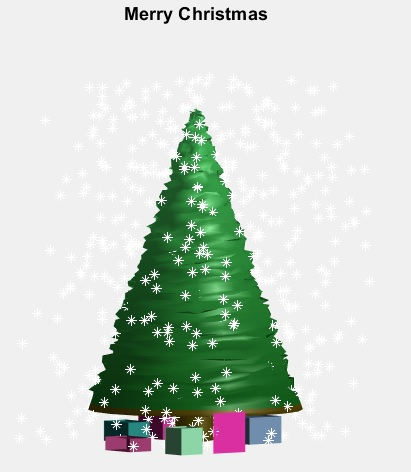## Conical tree

We start building a conical tree using the cylinder function of Matlab and defining the radious of the contour.

```totSlices = 101; %slices to define the contour
rad(2:10) = 1.5; % redefine trunk basis
```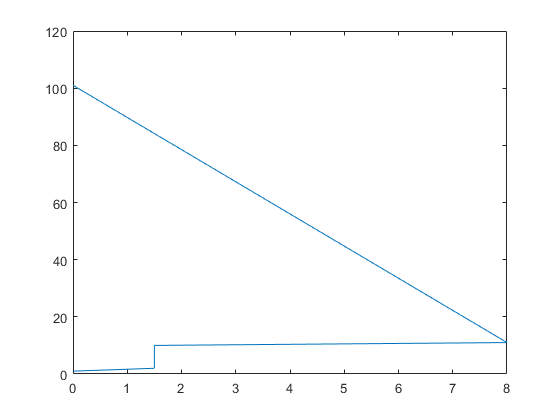## A Cone from a Cylinder

Matlab brings the cylinder function that becomes a cone if the radious is decreasing along the vertical direction. Each slice of the cylinder is a circle subdivided in 20 parts. That is, 21 points on the contour circle when you identify the extremal points. By default the cylinder heigth is 1.

```[X,Y,Z] = cylinder(rad); %produce a tree formed cylinder
Z = Z*25; %scale the heigth to have a higher tree (25m)
surf(X,Y,Z) %show the cone tree
view([-37.5,4]) %Change the 3D camera view to see better the 3D tree
```## Crease the tree surface:

Since the tree is very regular and smooth, we add some diffusion to the surface points of the tree to make it look more real.

```numContHorizt = 21;
numContVert = totSlices;
treeDiffusion = rand(numContVert,numContHorizt)-0.5; %some horizontal diffusion data computed for all points together

%add this diffusion to the grid points
for contH = 1:numContHorizt
for contV = 11:numContVert %starting above the trunk
%get the angle to always diffuse in direction of the radius
angle = atan(Y(contV,contH)/X(contV,contH));
%split the diffusion in the two coordinates, depending on the angle
X(contV,contH) = X(contV,contH)+cos(angle)*treeDiffusion(contV,contH);
Y(contV,contH) = Y(contV,contH)+sin(angle)*treeDiffusion(contV,contH);
%some small Vertical diffusion for each point
Z(contV,contH) = Z(contV,contH)+(rand-0.5)*0.5;
end
end
%draw the present tree
surfl(X,Y,Z,'light')
view([-37.5,4]) %Change the 3D camera view to see better the 3D tree
```## Color, view and format

Use as nice green color map (darker at the bottom, lighter at the top)

```r = (0.0430:(0.2061/50):0.2491)'; %red   component
g = (0.2969:(0.4012/50):0.6981)'; %green component
b = (0.0625:(0.2696/50):0.3321)'; %blue  component
map = [r,g,b]; %join in a color map table
for cnt = 1:5  %change the lower part to brown for the trunk
map(cnt,:) = [77,63,5]/265;
end

colormap(map); %set the map
view([-37.5,4])%Change the view to see a little more of the Actual 3D tree
lighting phong %some nice lighting
shading interp %remove grid and smoothen the surface color
axis equal     %takes care of display in the right proportion
axis([-10 10 -10 10 0 30]) %give some more axis space (for the snow later)
axis off %don't show axis
hold on  %remain open to draw the rest
title('Merry Christmas')
```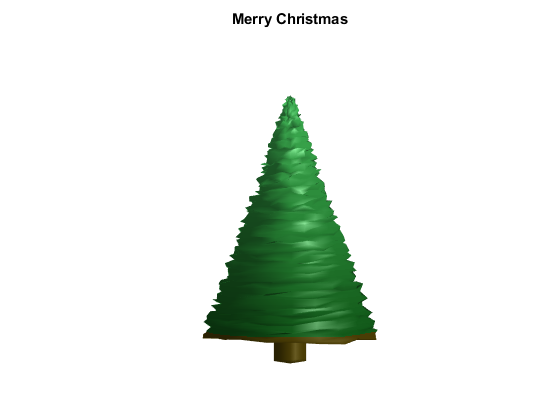## Presents

Draw some presents around the tree (each with random color), we use a function listed below that plots a present box according to the following parameters for translation and scale: drawPresent(px,py,pz,scalex,scaley,scalez)

```drawPresent(2,-4,0,3,3,2);
drawPresent(-4,3,0,2,3,1.5);
drawPresent(5,3,0,4,3,3);
drawPresent(-14,-5,0,6,3,1);
drawPresent(-9,-10,0,2,2,2);
drawPresent(0,4,0,4,3,3);
drawPresent(-6,-13,0,3,3,3);
```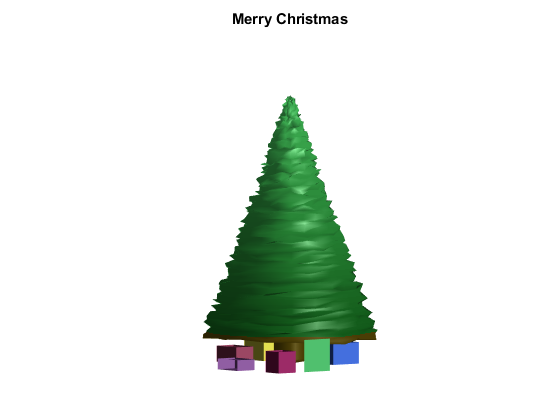## Setup Snow

Create some random 3D coordinates for the snow. Some snowflakes will end up inside the tree but just can't be seen then.

```snow = 800;     % number of snowflakes
snowX = (rand(snow,1)*25-12.5);
snowY = (rand(snow,1)*25-12.5);
snowZ = (rand(snow,1)*27);
plot3(snowX,snowY,snowZ,'w*') %plot coordinates as white snowflakes
hold off %the scene is done
```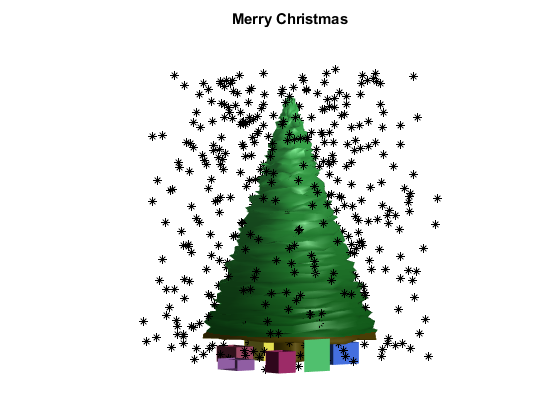## Function for Present Drawing

Draws a present box with the given coordinate + size in a random color Note: Given coordinates apply to the lower front + left corner of the present (the one closest to the viewer) as seen in the plot

```function drawPresent(dx,dy,dz,scalex,scaley,scalez)
% the standard present coordinates: [0,1]x[0,1]x[0,1] cube
presentX = [0.5 0.5 0.5 0.5 0.5; 0 1 1 0 0; 0 1 1 0 0; 0 1 1 0 0; 0.5 0.5 0.5 0.5 0.5];
presentY = [0.5 0.5 0.5 0.5 0.5; 0 0 1 1 0; 0 0 1 1 0; 0 0 1 1 0; 0.5 0.5 0.5 0.5 0.5];
presentZ = [0 0 0 0 0; 0 0 0 0 0; 0.5 0.5 0.5 0.5 0.5; 1 1 1 1 1; 1 1 1 1 1];
% scale present and move it to the right place and get the plot handle
myHandle = surf((presentX*scalex+dx),(presentY*scaley+dy), (presentZ*scalez+dz));
% draw some presents with random colors
randColorMap(:,:,1) = repmat(rand,[5,5]);%r component
randColorMap(:,:,2) = repmat(rand,[5,5]);%g component
randColorMap(:,:,3) = repmat(rand,[5,5]);%b component
% Assign colormap just to the plot handle object of the present, so the tree
% does not change color
set(myHandle,'CData',randColorMap)
end % of function
```

## Exercise:

Try to figure out how to get snow flakes folling down (....and record a video!!!)

Numerical Factory (2018)

```% https://es.mathworks.com/matlabcentral/fileexchange/22478-a-christmas-tree-plot
%
% Redistribution and use in source and binary forms, with or without
% modification, are permitted provided that the following conditions are
% met:
%
%     * Redistributions of source code must retain the above copyright
%       notice, this list of conditions and the following disclaimer.
%     * Redistributions in binary form must reproduce the above copyright
%       notice, this list of conditions and the following disclaimer in
%       the documentation and/or other materials provided with the distribution
%
% THIS SOFTWARE IS PROVIDED BY THE COPYRIGHT HOLDERS AND CONTRIBUTORS "AS IS"
% AND ANY EXPRESS OR IMPLIED WARRANTIES, INCLUDING, BUT NOT LIMITED TO, THE
% IMPLIED WARRANTIES OF MERCHANTABILITY AND FITNESS FOR A PARTICULAR PURPOSE
% ARE DISCLAIMED. IN NO EVENT SHALL THE COPYRIGHT OWNER OR CONTRIBUTORS BE
% LIABLE FOR ANY DIRECT, INDIRECT, INCIDENTAL, SPECIAL, EXEMPLARY, OR
% CONSEQUENTIAL DAMAGES (INCLUDING, BUT NOT LIMITED TO, PROCUREMENT OF
% SUBSTITUTE GOODS OR SERVICES; LOSS OF USE, DATA, OR PROFITS; OR BUSINESS
% INTERRUPTION) HOWEVER CAUSED AND ON ANY THEORY OF LIABILITY, WHETHER IN
% CONTRACT, STRICT LIABILITY, OR TORT (INCLUDING NEGLIGENCE OR OTHERWISE)
% ARISING IN ANY WAY OUT OF THE USE OF THIS SOFTWARE, EVEN IF ADVISED OF THE
% POSSIBILITY OF SUCH DAMAGE.
```